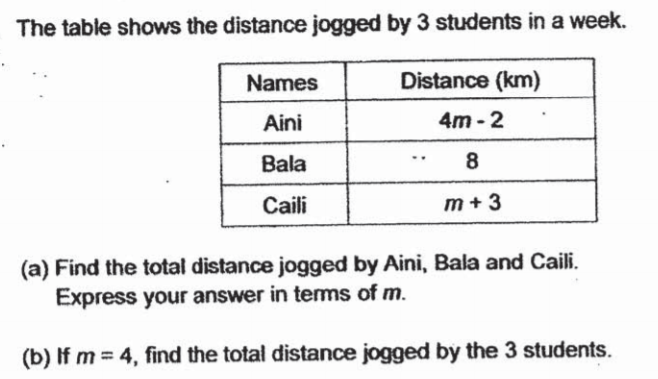### Still have math questions?

Algebra
QuestionThe table shows the distance iogqed by $$3$$ students in a week.

(a) Find the total distance jogged by Aini, Bala and Caili. Express your answer in terms of $$m$$ .

(b) If $$m = 4$$ , find the total distance jogged by the $$3$$ students.

$$\left. \begin{array} { l } { \text { a) } ( 4 m - 2 ) + 8 ( + m + 3 ) = ( 5 m + 9 ) k m } \\ { \text { b) } 4 ( 4 ) - 2 + 8 + 4 + 3 = 29 k m } \end{array} \right.$$